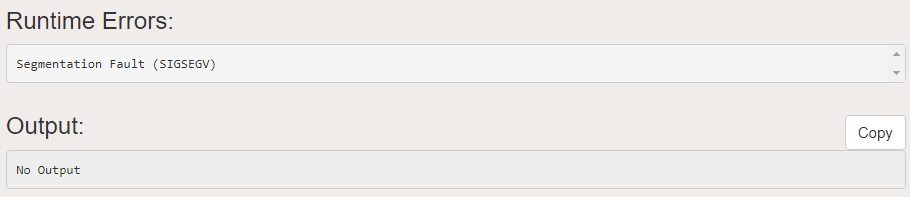GeeksforGeeks App
Open AppBrowser
Continue

# How to return a Pointer from a Function in C

Pointers in C programming language is a variable which is used to store the memory address of another variable. We can pass pointers to the function as well as return pointer from a function. But it is not recommended to return the address of a local variable outside the function as it goes out of scope after function returns.

Program 1:

The below program will give segmentation fault since ‘A’ was local to the function:

## C

 `// C program to illustrate the concept of``// returning pointer from a function``#include `` ` `// Function returning pointer``int``* fun()``{``    ``int` `A = 10;``    ``return` `(&A);``}`` ` `// Driver Code``int` `main()``{``    ``// Declare a pointer``    ``int``* p;`` ` `    ``// Function call``    ``p = fun();`` ` `    ``printf``(``"%p\n"``, p);``    ``printf``(``"%d\n"``, *p);``    ``return` `0;``}`

Output:
Below is the output of the above program:Explanation:

The main reason behind this scenario is that compiler always make a stack for a function call. As soon as the function exits the function stack also gets removed which causes the local variables of functions goes out of scope.

Static Variables have a property of preserving their value even after they are out of their scope. So to execute the concept of returning a pointer from function in C you must define the local variable as a static variable.

Program 2:

## C

 `// C program to illustrate the concept of``// returning pointer from a function``#include `` ` `// Function that returns pointer``int``* fun()``{``    ``// Declare a static integer``    ``static` `int` `A = 10;``    ``return` `(&A);``}`` ` `// Driver Code``int` `main()``{``    ``// Declare a pointer``    ``int``* p;`` ` `    ``// Function call``    ``p = fun();`` ` `    ``// Print Address``    ``printf``(``"%p\n"``, p);`` ` `    ``// Print value at the above address``    ``printf``(``"%d\n"``, *p);``    ``return` `0;``}`

Output:

```0x601038
10
```

My Personal Notes arrow_drop_up